Courses

# NCERT Solutions(Part- 4)- Squares and Square Roots Class 8 Notes | EduRev

## Class 8 : NCERT Solutions(Part- 4)- Squares and Square Roots Class 8 Notes | EduRev

The document NCERT Solutions(Part- 4)- Squares and Square Roots Class 8 Notes | EduRev is a part of the Class 8 Course Class 8 Mathematics by VP Classes.
All you need of Class 8 at this link: Class 8

Exercise 6.3

Question 1. What could be the possible ‘one’s’ digits of the square root of each of the following numbers?

(i) 9801 (ii) 99856 (iii) 998001 (iv) 657666025

Solution: The possible digit at one’s place of the square root of:

(i) 9801 can be 1 or 9.

[∵ 1 *1 = 1 and 9 * 9 = 81]

(ii) 99856 can be 4 or 6.

[∵ 4 * 4 = 16 and 6 * 6 = 36]

(iii) 998001 can be 1 or 9.

[∵ 1 *1 = 1 and 9 * 9 = 81]

(iv) 657666025 can be 5.

[∵ 5 * 5 = 25]

Question 2. Without doing any calculation, find the numbers which are surely not perfect squares.

(i) 152 (ii) 257 (iii) 408 (iv) 441

Solution: We know that the ending digit of perfect square is 0, 1, 4, 5, 6, and 9.

∴ A number ending in 2, 3, 7 or 8 can never be a perfect square.

(i) 153, cannot be a perfect square.
(ii) 257, cannot be a perfect square.
(iii) 408, cannot be a perfect square.
(iv) 441, can be a perfect square.

Thus, (i) 153, (ii) 257 and (iii) 408 are surely not perfect squares.

Question 3. Find the square roots of 100 and 169 by the method of repeated subtraction.

Solution:

(i)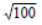We have

100 – 1 = 99  99 – 3 = 96  96 – 5 = 91
91 – 7 = 84    84 – 9 = 75  75 – 11 = 64
64 – 13 = 51  51 – 15 = 36  36 – 17 = 19
19 – 19 = 0

∵ We reach at 0 by successive subtraction of 10 odd numbers.

∴= 10.

(ii)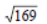We have

169 – 1 = 168  168 – 3 = 165  165 – 5 = 160
160 – 7 = 153  153 – 9 = 144  144 – 11 = 133
133 – 13 = 120  120 – 15 = 105  105 – 17 = 88
88 – 19 = 69  69 – 21 = 48  48 – 23 = 25
25 – 25 = 0

∵ We reach at 0 by successive subtraction of 13 odd numbers.= 13.

Question 4. Find the square roots of the following numbers by the Prime Factorisation Method.

(i) 729 (ii) 400 (iii) 1764 (iv) 4096 (v) 7744
(vi) 9604 (vii) 5929 (viii) 9216 (ix) 529 (x) 8100

Solution:

(i) We have

729 = 3 * 3 * 3 * 3 * 3 * 3

∴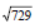= 3 * 3 * 3 = 27

Thus, the square root of 729 = 27.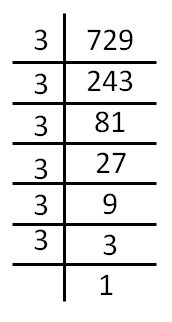(ii) We have

400 = 2 * 2 * 2 * 2 * 5 * 5

∴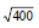= 2 * 2 * 5 = 20

Thus, the square root of 400 is 20.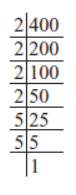(iii) We have

1764 = 2 * 2 * 3 * 3 * 7 * 7

∴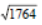= 2 * 3 * 7 = 42

∴ The square root of 1764 is 42.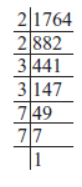(iv) We have

4096 = 2 * 2 * 2 * 2 * 2 * 2 * 2 * 2 * 2 * 2 * 2 * 2

∴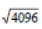= 2 * 2 * 2 * 2 * 2 * 2 = 64

Thus, the square root of 4096 is 64.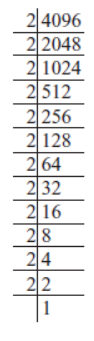(v) We have

7744 = 2 * 2 * 2 * 2 * 2 * 2 * 11 * 11

∴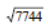= 2 * 2 * 2 * 11 = 88

Thus, the square root of 7744 is 88.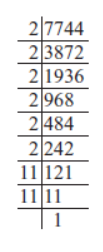(vi) We have

9604 = 2 * 2 * 7 * 7 * 7 * 7

∴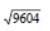= 2 * 7 * 7 = 98

Thus, the square root of 9604 is 98.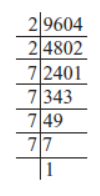(vii) We have

5929 = 7 * 7 * 11 * 11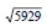= 7 * 11 = 77

Thus, the square root of 5929 is 77.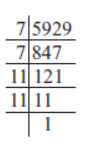(viii) We have

9216 = 2 * 2 * 2 * 2 * 2 * 2 * 2 * 2 * 2 * 2 * 3 * 3
∴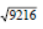= 2 * 2 * 2 * 2 * 2 * 3 = 96

∴ The square root of 9216 is 96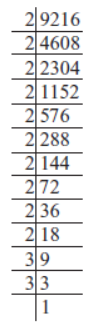(ix) We have:

529 = 23 * 23

∴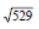= 23

Thus, the square root of 529 is 23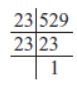(x) We have

8100 = 2 * 2 * 3 * 3 * 3 * 3 * 5 * 5

∴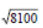= 2 * 3 * 3 * 5 = 90

Thus, the square root of 8100 is 90.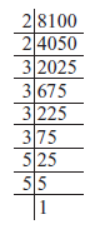Question 5. For each of the following numbers, find the smallest whole number by which it should be multiplied so as to get a perfect square number. Also find the square root of the square number so obtained.

(i) 252 (ii) 180 (iii) 1008 (iv) 2028 (v) 1458 (vi) 768

Solution:

(i) We have

252 = 2 * 2 * 3 * 3 * 7

∵ The prime factor 7 has no pair.

∴  * 7 = [2 * 2 * 3 * 3 * 7] * 7

or 1764 = 2 * 2 * 3 * 3 * 7 * 7

and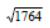= 2 * 3 * 7 = 42

Thus, the required smallest whole number = 7

Also, the square root of 1764 is 42.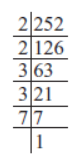(ii) We have

180 = 2 * 2 * 3 * 3 * 5

∵ The prime factor 5 has no pair.

∴  * 5 = [2 * 2 * 3 * 3 * 5] * 5

or  900 = 2 * 2 * 3 * 3 * 5 * 5

or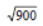= 2 * 3 * 5 = 30

Thus, the required smallest whole number = 5

Also, the square root of 900 is 30.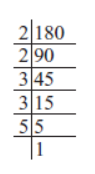(iii) We have

1008 = 2 * 2 * 2 * 2 * 3 * 3 * 7

∵ The prime factor 7 has no pair.

∴  * 7 = [2 * 2 * 2 * 2 * 3 * 3 * 7] * 7

or 7056 = 2 * 2 * 2 * 2 * 3 * 3 * 7 * 7

or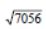= 2 * 2 * 3 * 7 = 84

Thus, the required smallest whole number = 7

Also the square root of 7056 is 84.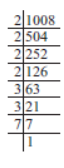(iv) We have

2028 = 2 * 2 * 3 * 13 * 13

∵ The prime factor 3 has no pair.

∴  * 3 = [2 * 2 * 3 * 13 * 13] * 3

or 6084 = 2 * 2 * 3 * 3 * 13 * 13

or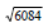= 2 * 3 * 13 = 78

Thus, the smallest whole number = 3 and= 78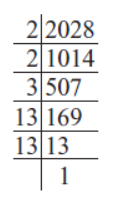(v) We have

1458 = 2 * 3 * 3 * 3 * 3 * 3 * 3

∵ 2 has no pair.

∴  * 2 = [2 * 3 * 3 * 3 * 3 * 3 * 3] * 2

or 2916 = 2 * 2 * 3 * 3 * 3 * 3 * 3 * 3

or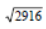= 2 * 3 * 3 * 3 = 54

Thus, the required smallest whole number = 2, and= 54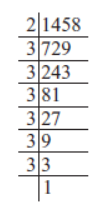(vi) We have

768 = 2 * 2 * 2 * 2 * 2 * 2 * 2 * 2 * 3

∵ Prime factor 3 has no pair.

∴  * 3 = [2 * 2 * 2 * 2 * 2 * 2 * 2 * 2 * 3] * 3

2304 = 2 * 2 * 2 * 2 * 2 * 2 * 2 * 2 * 3 * 3

or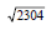= 2 * 2 * 2 * 2 * 3 = 48

Thus, the smallest whole number = 3 and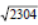= 48.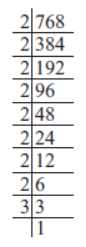Question 6. For each of the following numbers, find the smallest whole number by which it should be divided so as to get a perfect square. Also find the square root of the square number so obtained.

(i) 252 (ii) 2925 (iii) 396 (iv) 2645 (v) 2800 (vi) 1620

Solution:

(i) 252

We have

252 = 2 * 2 * 3 * 3 * 7

∵ The prime factor 7 is unpaired, so the given number should be divided by 7.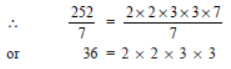Thus, 36 is a perfect square. and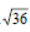= 2 * 3 = 6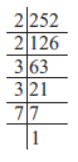(ii) 2925

We have

2925 = 3 * 3 * 5 * 5 * 13

∵ The prime factor 13 is unpaired, so the given number should be divided by 13.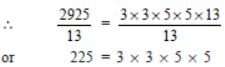Thus, 225 is a perfect square. and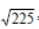= 3 * 5 = 15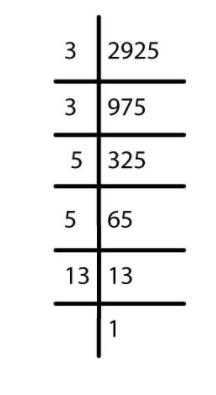(iii) 396

We have

396 = 2 * 2 * 3 * 3 * 11

As the prime factor 11 is unpaired.

∴  Dividing the given number by 11,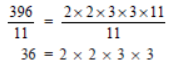∴ 36 is a perfect square. and,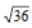= 2 * 3 = 6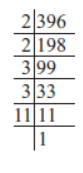(iv) 2645

We have

2645 = 5 * 23 * 23

∵ The prime factor 5 is unpaired.

∴ Dividing the given number by 5, we have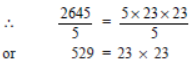Thus, 529 is a perfect square, and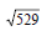= 23.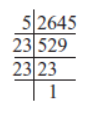(v) 2800

We have

2800 = 2 * 2 * 2 * 2 * 5 * 5 * 7

Since, 7 is unpaired.

∴ The given number should be divided by 7.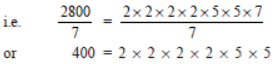which is a perfect square.

∴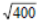= 2 * 2 * 5 = 20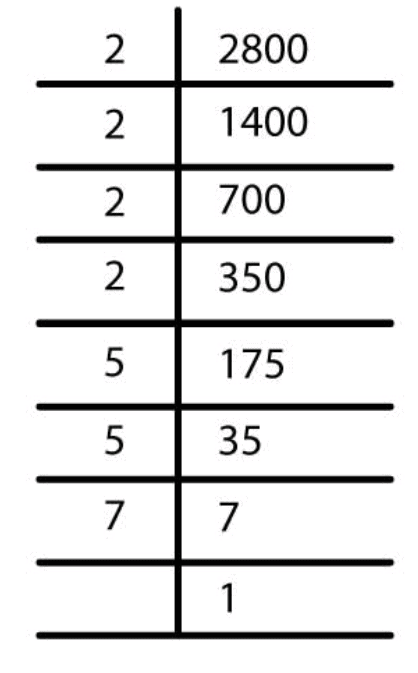(vi) 1620

We have

1620 = 2 * 2 * 3 * 3 * 3 * 3 * 5

∵ The prime factor 5 is unpaired.

∴ Dividing the given number by 5, we have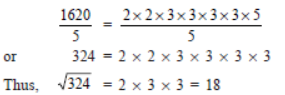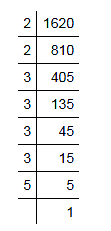Question 7. The students of Class VIII of a school donated Rs 2401 in all, for Prime Minister’s National Relief Fund. Each student donated as many rupees as the number of students in the class. Find the number of students in the class.

Solution: Let the number of students = x

∴  Each student donated Rs x.

Total amount donated by the class = Rs x * x = Rs x2

Thus, x2 = 2401

or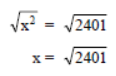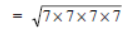= 7 * 7 = 49

∴ The number of students in the class = 49.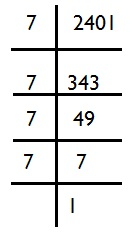Question 8. 2025 plants are to be planted in a garden in such a way that each row contains as many plants as the number of rows. Find the number of row and the number of plants in each row.

Solution: Let the number of rows =x

∴  Number of plants is a row = x

So, the number of plants to be planted = x * x = x2

x2 = 2025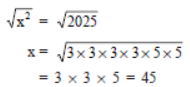Thus, the required number of rows = 45

Also, number of plants in a row = 45.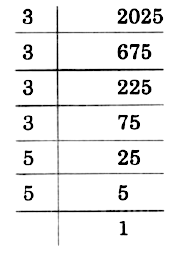Question 9. Find the smallest square number that is divisible by each of the numbers 4, 9 and 10.

Solution: We know that LCM is the smallest number divisible by all its factors.

Since, LCM of 4, 9 and 10 = 2 * 2 * 9 * 5 = 180

But 180 is not a perfect square.

Again,

180 = 2 * 2 * 3 * 3 * 5  [∵ 9 = 3 * 3]

∵ It has 5 as unpaired.

∴  * 5 = [2 * 2 * 3 * 3 * 5] * 5

or 900 = 2 * 2 * 3 * 3 * 5 * 5

∵ All the prime factors of 900 are paired.

∴ 900 is a perfect square.

Thus, the required number = 900.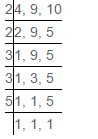Question 10. Find the smallest square number that is divisible by each of the numbers 8, 15 and 20

Solution: The smallest number divisible by 8, 15 and 20 is their LCM.

We have LCM = 2 * 2 * 5 * 2 * 3 = 120

But 120 is not a square number.

Now, to make it a perfect square, we have

120 = 2 * 2 * 2 * 3 * 5

or  * 2 * 3 * 5 = [2 * 2 * 2 * 3 * 5] * 2 * 3 * 5

or 3600 = 2 * 2 * 2 * 2 * 3 * 3 * 5 * 5

All factors of 3600 are paired. Therefore, 3600 is a perfect squared.

∴ The required number = 3600.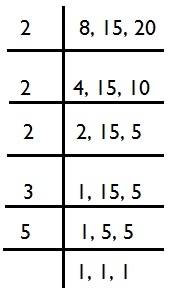Offer running on EduRev: Apply code STAYHOME200 to get INR 200 off on our premium plan EduRev Infinity!

89 docs|16 tests

,

,

,

,

,

,

,

,

,

,

,

,

,

,

,

,

,

,

,

,

,

;